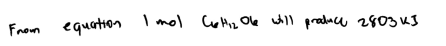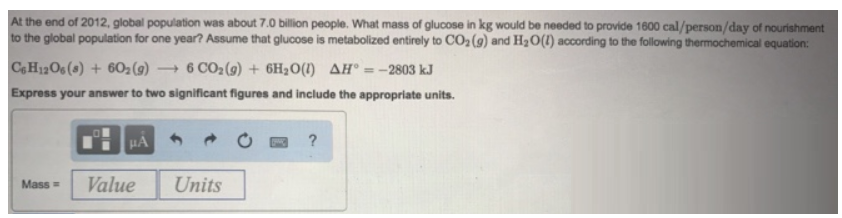# Problem: At the end of 2012, global population was about 7.0 billion people. What mass of glucose in kg would be needed to provide 1600 cal/person/day of nourishment to the global population for one year? Assume that glucose is metabolized entirely to CO2 (g) and H2O(l) according to the following thermochemical equation: C6H12O6(s)+ 6O2(g) → 6CO2(g) + 6H2O(l)         ΔH° = 2803 kJ Express your answer to two significant figures and include the appropriate units.

###### FREE Expert Solution83% (341 ratings)###### Problem Details

At the end of 2012, global population was about 7.0 billion people. What mass of glucose in kg would be needed to provide 1600 cal/person/day of nourishment to the global population for one year? Assume that glucose is metabolized entirely to CO2 (g) and H2O(l) according to the following thermochemical equation:

C6H12O6(s)+ 6O2(g) → 6CO2(g) + 6H2O(l)         ΔH° = 2803 kJ

Express your answer to two significant figures and include the appropriate units.